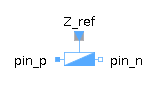Fixed Frame - MapleSim Help

Fixed Frame

A stationary frame with a fixed displacement and orientation relative to groundDescription A Fixed Frame is attached rigidly to the mechanical ground.  The frame of the component, which is shown in the diagram below as ${x}_{2}{y}_{2}$${z}_{2}$, may be rotated and offset with respect to the ground frame, which is represented by ${x}_{1}{y}_{1}$${z}_{1}$in the diagram. The translation of the end frame, ${x}_{2}{y}_{2}$relative to the ground frame, ${x}_{1}{y}_{1}$${{z}_{1}}_{}$, is represented by the vector, r , resolved into its three components in the ${x}_{1}{y}_{1}$${{z}_{1}}_{}$ frame.  The rotation of ${x}_{2}{y}_{2}$${z}_{2}$, relative to ${x}_{1}{y}_{1}$${z}_{1}$, can be represented by three Euler angles, which represent three subsequent rotations from the ground frame to the component frame, or by the rotation matrix ${\stackrel{&conjugate0;}{R}}_{}$ (  ${\stackrel{&conjugate0;}{R}}_{}\cdot {\stackrel{&conjugate0;}{r}}_{2}$ ).Connections

 Name Description ${\mathrm{frame}}_{b}$ Outboard frameParameters

 Symbol Default Units Description Modelica ID ${\stackrel{&conjugate0;}{r}}_{\mathrm{XYZ}}$ $\left[\begin{array}{ccc}0& 0& 0\end{array}\right]$ $m$ The x,y,z offset of the outboard frame with respect to ground. InitPos ${\mathrm{Type}}_{\mathrm{R}}$ Euler Angles Indicates whether the rotation matrix, ${\stackrel{&conjugate0;}{R}}_{}$, or the rotation sequence, ${\stackrel{&conjugate0;}{\theta }}_{\zeta ,\eta ,\xi }$, is used to express the orientation of the outboard frame with respect to ground. RSelect $\stackrel{}{\left[R\right]}$ $\left[\begin{array}{ccc}1& 0& 0\\ 0& 1& 0\\ 0& 0& 1\end{array}\right]$ Rotation matrix that transforms a vector, expressed in the local frame to the inertial frame. RMat ${\mathrm{Type}}_{\mathrm{θ}}$ $\left[\begin{array}{ccc}1& 2& 3\end{array}\right]$ Indicates the sequence of subsequent rotations describing the orientation of the outboard frame with respect to ground.  For example, $\left[1,2,3\right]$ refers to sequential rotations about the x, then y, and then the z axis (123 - Euler angles). RotType ${\stackrel{&conjugate0;}{\theta }}_{\zeta ,\eta ,\xi }$ $\left[\begin{array}{ccc}0& 0& 0\end{array}\right]$ $\mathrm{rad}$ Rotations about the axes selected in ${\mathrm{Type}}_{\mathrm{\theta }}.$ InitAngSee Also